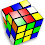## Friday, May 09, 2008

### Mothers' Day Puzzle for all our Supermoms

Take the following mathematical equation:

MOM2 = AMAZON

Can you replace each letter with a different digit {0 to 9} so that the equation makes sense? The letter will represent that digit everywhere the letter appears.

1.Should I assume that the LH side of the equation is (MOM)squared, or M*0*Msquared??

2.3.Come on... it's not that hard. And to clarify once again, that is the letter 'O' which can be any digit, not just zero.

4.Well, I have the answer. But is there a less tedious solution than working through the multiplication digit by digit?

5.Obviously if M and O are set, then the other values are decided. The smallest six-digit square with different digits is 323^2. The largest is 989^2. There are also things you can notice like M can't be 5 or 6, so that narrows things down.

For NPR puzzle posts, don't post the answer or any hints that could lead to the answer before the deadline (usually Thursday at 3pm ET). If you know the answer, submit it to NPR, but don't give it away here.

You may provide indirect hints to the answer to show you know it, but make sure they don't assist with solving. You can openly discuss your hints and the answer after the deadline. Thank you.